Latest topics
» Homework listWed Mar 23, 2011 12:26 am by AdminTue Mar 22, 2011 9:02 pm by AdminTue Mar 22, 2011 7:59 am by fergus

» Find k - quadratics problemWed Mar 16, 2011 12:40 am by Admin

» S1- probability questionMon Mar 07, 2011 8:54 pm by AdminMon Mar 07, 2011 8:31 pm by Admin

» surds problemSun Feb 27, 2011 7:02 am by fergus

» Solving equationsMon Feb 21, 2011 7:35 pm by Admin

» Circle theorem questionSun Feb 06, 2011 2:09 am by Admin

Poll
Who is online?
In total there is 1 user online :: 0 Registered, 0 Hidden and 1 Guest

None

Most users ever online was 69 on Wed Jul 03, 2019 10:37 am

# Fibonacci Sequence and the Golden ratio##Fibonacci Sequence and the Golden ratio

1.What is the Fibonacci sequence and does it occur in real life- where?
2. What is the golden ratio?
3. What is the connection between the Fibonacci sequence and the golden ratio?

enjoy##Fibonacci Sequence

Fibonacci Sequence
Alright, so the Fibonacci sequence is when you have the numbers 0,1,1,2,3,5,8,13,21,34,55,89 and so on.

With the Fibonacci sequence you start with zero and one, then you add the two numbers to make the number after that.then repeat with the last number and the number you got. For example 0+1=1 Right? So we put one next to the first two numbers. Then 1+1=2 Right? So we add that next to the two ones. This is the Fibonacci sequence.

Golden ratio
The golden ratio is often encountered when you are taking the ratio of distances.

If you divide a line into two parts so that the longer part divided by the smaller part IS ALSO EQUAL TO the whole length divided by the longer part. Then you will have the golden ratio. This is very confusing and it took me half an hour to figure it out so my method of explanation may not be the bestThe Connection

Any power of the golden ratio can be ultimately reduced to the sum of an integer and an integer multiple of the golden ratio. Actually, All of the numbers turn out to be Fibonacci numbers. Here is the connection between the Two.

Small note: If i get this sticker, then that will make five.

It also took me a very long time to right that up##goo start ...

Well done. Thats a good start. Can somebody add to this...?

##golden rectangle ???

i researched what the link could be and alot of the answers i found had something to do with the golden rectangle as well... what you do is get a square thats sides are 1 and 1, find the longest side and add a square of that size to form a rectangle! so from a square with 1 and 1, there would be a rectangle of 1 and 2, then another of 3 and 2 and then of 3 and 5 and so on.. heres another explanaition:
###
# # 1 by 1, so we add. 1 by 1 to get...
###

######
# ## # Now it is 2 by 1, so we add 2 by 2 to get......
######

######
# ## #
######
######
# # Now it is 2 by 3, so we add a 3 by 3 to get.......
# #
# #
# #
######

###############
# ## ## #
####### #
# ## #
# ## # Now it is 3 by 5, so we would add a 5 by 5 square.
# ## #
# ## #
###############
oh by the way, a golden rectangle is a rectangle whose lengths are in the golden ratio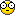##Too many Subjects to list....

Sorry Emma, but I don't really get what your saying...
Can you make it a little simpler?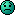##I gottit!

Ok so first you have to keep dividing the numbers in the Fibonacci sequence by the ones before it so:
1/1 = 1
2/1 = 2
3/2 = 1.5
5/3 = 1.666666666
8/5 = 1.6
13/8 = 1.625
21/13 = 1.615384615
34/21 = 1.619047619
55/34 = 1.617647059
89/55 = 1.618181818 -THE GOLDEN RATIO
....................... ....................... ......................##Excellent

Well done guys! this is an excellent start... Emma has found the connection... can somebody add a picture to do witht the golden ratio or the fibonacci sequence??

##the picture

The picture of the connection of the Fibonacci Sequence and the Golden Ratio is simply a spiral. The link between the Fibonacci Sequence and the Golden Ratio is because they all spiral outwards. The Fibonacci Sequence is keeps on spiraling outwards until it reaches the Golden Ratio number 1.618. That is the link. The picture Mr. Safdar asked us is shown below: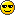##Fibonacci Sequence and the golden ratio

what is...
Fibonacci sequence...:the number has invented from Italian mathematician Fibonnaci.There are-0,1,1,2,3,5,8,13,21,34,55.....
Golden ratio...:1.618 when we divede by any numbers before it like Maya said, we get a golden ratio.
The number 1.618 is everywhere. Nature, our body, buildings...I don't know how to show you the video but in YouTube <Golden Ratio in Human Body> is very interesting!!

##Fibonnaratio

The Golden Ratio is approximately 1.618 and is the ratio of the length to the width of the Golden Rectangle. I have tried to cut and paste a picture of the Golden Rectangle to show the ratios of length to width, but it didn't work.The Golden Ratio is the ratio for measurements of many things in the human body. Artists and scientists have used this in their work to represent (draw, paint or sculpt) and to understand the human body. For example - the ratio between the width of my mum's nose (4cm) and the length of her lips (6.5cm) = 1.625cm. The ratio is the same for the length of the fingers to the elbow over the lenght of the wrist to the elbow. It is also the same for the length of the face over the width of the face. The length of the two front teeth over their width. It just keeps going. It's crazy!!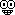Watch this amazing video - https://www.youtube.com/watch?v=085KSyQVb-U

The Fibonnaci sequence is a sequence of numbers where the sum of the last two numbers equals the next number. Example: 0,1 = 0+1=1, 1+1 = 2, so the sequence is: 0,1,1,2, 3, 5 and so on. The Golden Ratio and the Fibonnaci sequence are related because when you divide the Fibonacci numbers in their order to create a ratio between one and the next (for example: 3 divided by 5 and 5 divided by 8 ), you get close to and then only up to (but never over) the Golden Ratio. So you get:

1 divided by 1 = 1
1 divided by 2 = .5
3 divided by 2 = 1.5
5 divided by 3 = 1.6
8 divided by 5 = 1.6
13 divided by 8 = 1.6
21 divided by 13 = 1.6
34 divided by 21 = 1.6
55 divided by 34 = 1.6
89 divided by 55 = 1.6

This is amazingly the approximate value of the Golden Ratio. How is it possible?!Last edited by Daraius Kirk on Fri Nov 05, 2010 10:55 pm; edited 1 time in total (Reason for editing : adding information on the human body)

##Picture.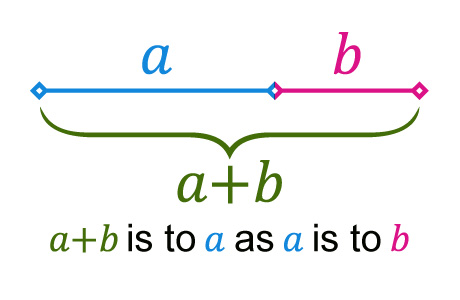Yo, so here we got the Golden Ratio, Fibonacci sequence and the Golden spiral.

##D'aaaw!

I Can't believe i Didn't notice the division thing.
Nothings going right for me..If you want any other pictures inserted, ask the man##Nice

Well done guys! I'm impressed! Meejin - when you're typing if you click on the movie link you should be able show us the youtube clip

##Re: Fibonacci Sequence and the Golden ratio

ok ayaan... You know that picture that you put of a spiral? The big rectangle behind it (cut up into little rectangles and a square) is the golden rectangle. The golden rectangle is a rectangle whose sides are the golden ratio. To make it you have to start with a square which is 1 by 1. Then you take the longest side and add it to the other side to make one side of your new rectangle(so now you would have to add 1 to 2)... Now you make a rectangle with 2 (as 1+1=2) and 1(because you keep the smallest length). The next rectangle should be 2 by 3. You keep on going and the numbers should be the same as the ones from the fibonacci system-1,1,2,3,5,8,13,21 ect. When you put these rectangles and square together they form a golden rectangle. I hope I explained it better!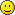##Re: Fibonacci Sequence and the Golden ratio

how do you put pictures?! help!##Help!

I also need help in how to insert a picture as Emma has said already. How do you do it. I tried over a million times but I could not bring it. Please help!!!!!!!##Thanks!

Thanks alot Emma, I get it now.
About pictures, first you go to the image inserter where you want to post, the from your image, select "Copy image location" Then, in the bar that comes up, paste it.
Hopefully this'll help!

P.S:Dont you just love my avatar, the cats all KERCHOOW! NIIIIIINNNNJAAAA!

##Still more help

I still don't get how to insert a picture. Your explaination was to complicated.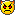I do like your avatar Ayaan, it cool.

##!!!!!

I don't know how to put pictures....I tried to copy but no...and I tried the thing on the top (images) but.....help!

##Re: Fibonacci Sequence and the Golden ratio

Thank you Kal.

Images.. Sigh.

When you post, you see a little button that says images. I presume thou shalt use google images? Cause this wont work for anything else. So then you right click on the data that you wish to transfer onto the post, select the button that says "copy image location"
Paste it into the image link, Huzzah. You have achieved the picture. This deserves a picture. But I will insert something about maths.##....

I kind of got Ayaan's explaining...but my computer isnot in English so...I don't know which buttons I should click! any other easy way??

##Re: Fibonacci Sequence and the Golden ratio

Meejin, try using google translate. Maybe it can help you out, if that doesnt work then I'm sorry.

##Re: Fibonacci Sequence and the Golden ratio